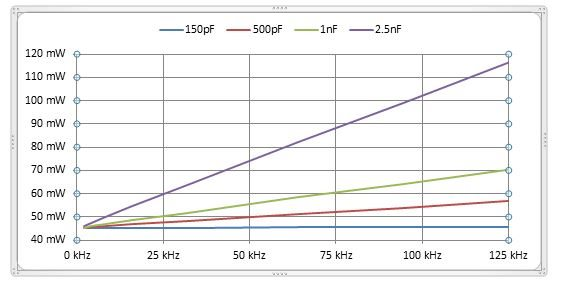# Active RS-232 power consumption: Why isn’t it in the data sheet?

System designers will often not find active power consumption mentioned in a typical data sheet. Many RS-232 interface device data sheets only specify supply current for unloaded and shutdown setups. However, an RS-232 device is only useful for communications when connected to a remote RS-232 device. The data cable’s capacitance and remote receiver’s resistance are loads to the local RS-232 device that increase power consumption. While most new RS-232 devices will have at least one active current or power specification, there are many with none.

The active power consumption is the sum of the power consumed by the load and the power lost in the device. The first step is to calculate the load power for the receiver resistor and cable capacitance. Equation 1 is the remote receiver resistor power formula, expressed as the number of channels multiplied by driver voltage squared divided by receiver resistance.

N x V2 / R                                (1)

Equation 2 is the data cable’s power consumption expressed as driver peak-to-peak voltage squared multiplied by frequency and capacitance. The number of local RS-232 drivers doesn’t matter because only one driver toggles at a time.

F x C x (2 x V) 2                      (2)

The maximum frequency for a constantly toggling RS-232 data stream is half of the BAUD rate. The frequency for an arbitrary data stream is thirty percent of the BAUD rate.

The total load power is the sum of the resistive power (equation 1) and capacitive power (equation 2).

P = N x V2 / R + F x C x (2 x V) 2

For devices that don’t have capacitor- or inductive-charge pumps, the supply current needed for the load is the same as the load current. Supply and output current are directly proportional, just like a linear voltage regulator.

Therefore, you will need to convert the load power into a load current. Load current is load power divided by the driver voltage.

I = (N x V2 / R + F x C x (2 x V) 2) / V

This simplifies into equation 3, active load current.

I = N x V / R + 4 x F x C x V                   (3)

Use the active load current, which is same as supply current, to calculate supply power consumed as a result of the load. Add the no load power to get the total system device power

Here are two examples of how to calculate active supply power.

Example 1

The GD75232 transceiver has three drivers and five receivers. VDD = 9V, VSS = -9V and VCC = 5V. The maximum supply currents are 15mA, 15mA and 30mA. The maximum no-load power is 9V x 15mA + -9V x -15mA + 5V x 30mA = 420mW. This is a static (no load) power.

Data stream is 120k-baud alternating bit pattern, the cable capacitance is 2500pF and the remote receiver is 3 kilohms (kohms) resistance. RS-232 driver voltage is 7.5V.

Calculate the load current substituting example parameters into equation 3.

I = 3 channels x 7.5V/ 3000 kohms + 4 x 120kbps/2 x 2500pF x 7.5V = 12mA

Because this current comes from VDD or VSS, the supply power needed to support the load is 9V x 12mA = 108mW.

Total power is no-load (static) power – 420mW – plus the active power – 108mW – for a total power of 528mW.

Example 2

The TRS3232E multichannel RS-232 line driver/receiver has two drivers and two receivers. VCC = 5V. No-load ICC is 1mA maximum.

This device has a capacitor-charge pump that boosts and inverts voltage at the expense of current. If the data sheet had two specified loads, you could calculate the charge-pump efficiency, but it does not. In this case, you must measure active current empirically. Power consumption varies with frequency of the data stream and cable capacitance as well as receiver input resistance. See an example below:Figure 1: TRS3232E power consumption vs. frequency, resistance 3 kohms/channel

Early TTL-based RS-232 devices did not have active-current specifications; that was OK because the active power was easy to calculate given the triple power-supply topology. Later single-supply charge pump RS-232 devices continued the “no specification for active power on the data sheet” mentality, but that’s a mistake, because you can’t calculate power from the data sheet alone. Visit the TI E2E™ community RS-232 forum for help wherever the data sheet doesn’t provide sufficient information.